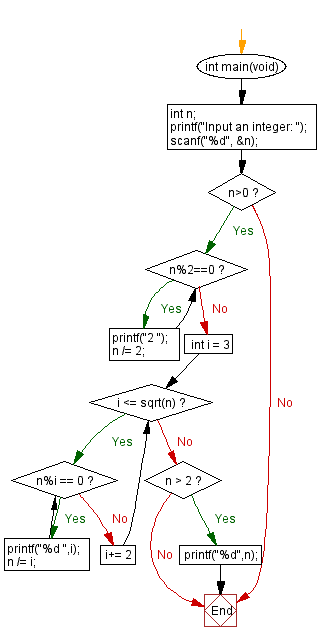﻿ C - Find all prime factors of an integer

# C Programming: Find all prime factors of a given integer

## C Programming Mathematics: Exercise-35 with Solution

Write a C program that accepts an integer and find all prime factors of the integer.

Prime factors of a number are those factors that are prime numbers. 2 and 4 are factors of 4, where 2 is considered the prime factor.

Test Data:
(77) -> 7, 11
(12) -> 2, 2, 3
(45) -> 3, 3, 5

Sample Solution:

C Code:

``````#include <stdio.h>
#include <math.h>

int main(void) {
int n;
printf("Input an integer: ");
scanf("%d", &n);
if (n>0)
{
while (n%2==0)
{
printf("2 ");
n /= 2;
}
for (int i = 3; i <= sqrt(n); i+= 2)
{
while (n%i == 0)
{
printf("%d ",i);
n /= i;
}
}
if (n > 2)
printf("%d",n);
}
}

```
```

Sample Output:

```Input an integer: 78
2 3 13

```

Flowchart:C Programming Code Editor:

Improve this sample solution and post your code through Disqus.

What is the difficulty level of this exercise?

Test your Programming skills with w3resource's quiz.

﻿